# Thread: Integration using substitution

1. ## Integration using substitution

(x^3) (x^2 +1)^(1/2)

can anyone tell me what i should substitute here?

2.Originally Posted by mag6(x^3) (x^2 +1)^(1/2)

can anyone tell me what i should substitute here?
$\displaystyle \int{x^3\sqrt{x^2 + 1}\,dx}$.

Try $\displaystyle x = \sinh{t}$ so that $\displaystyle dx = \cosh{t}\,dt$.

The integral becomes

$\displaystyle \int{\sinh^3{t}\sqrt{\sinh^2{t} + 1}\,\cosh{t}\,dt}$

$\displaystyle = \int{\sinh^3{t}\cosh{t}\sqrt{\cosh^2{t}}\,dt}$

$\displaystyle = \int{\sinh^3{t}\cosh^2{t}\,dt}$

$\displaystyle = \int{\sinh^2{t}\cosh^2{t}\sinh{t}\,dt}$

$\displaystyle = \int{(\cosh^2{t} - 1)\cosh^2{t}\sinh{t}\,dt}$

$\displaystyle = \int{(\cosh^4{t} - \cosh^2{t})\sinh{t}\,dt}$.

Now make the substitution $\displaystyle u = \cosh{t}$ so that $\displaystyle du = \sinh{t}\,dt$.

The integral becomes

$\displaystyle \int{u^4 - u^2\,du}$

$\displaystyle = \frac{u^5}{5} - \frac{u^3}{3} + C$

$\displaystyle = \frac{\cosh^5{t}}{5} - \frac{\cosh^3{t}}{3} + C$

$\displaystyle = \frac{(\cosh^2{t})^{\frac{5}{2}}}{5} - \frac{(\cosh^2{t})^{\frac{3}{2}}}{3} + C$

$\displaystyle = \frac{(\sinh^2{t} + 1)^{\frac{5}{2}}}{5} - \frac{(\sinh^2{t} + 1)^{\frac{3}{2}}}{3} + C$

$\displaystyle = \frac{(x^2 + 1)^{\frac{5}{2}}}{5} - \frac{(x^2 + 1)^{\frac{3}{2}}}{3} + C$

$\displaystyle = \frac{\sqrt{(x^2 + 1)^5}}{5} - \frac{\sqrt{(x^2 + 1)^3}}{3} + C$.

3. Another way to do it:

$\displaystyle \int x^3\sqrt{x^2+ 1} dx= \int (x^2)\sqrt{x^2+ 1} (xdx)$

Let $\displaystyle u= x^2+ 1$ so du= 2x dx and xdx= (1/2)du.

Also, x^2= u- 1.

Now the integral is $\displaystyle \frac{1}{2}\int (u- 1)u^{1/2}du= \frac{1}{2}\int u^{3/2}- u^{1/2} du$

$\displaystyle = \frac{1}{2}\left(\frac{2}{5}u^{5/2}- \frac{2}{3}u^{3/2}\right)+ C$
$\displaystyle = \frac{1}{5}(x^2+ 1)^{5/2}- \frac{1}{3}(x^2+ 1)^{3/2}+ C$

That is, of course, the same as Prove It's answer.

4. And another...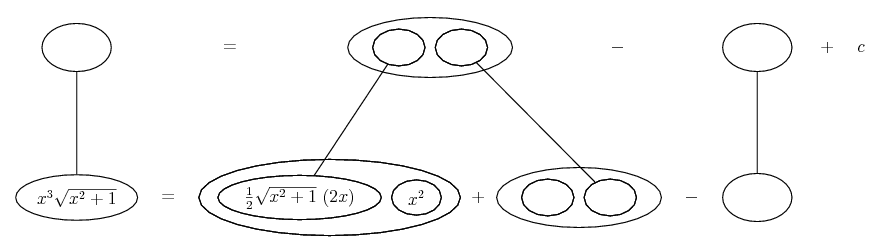... where... is the product rule (straight lines differentiating downwards), so that...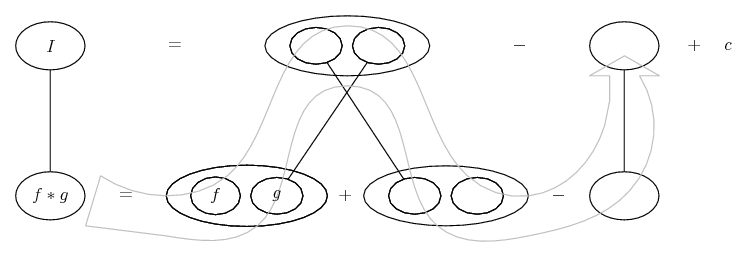is integration by parts, but (I hope) a lazy version where choosing legs crossed or un-crossed does for assigning u du and v dv.

Spoiler: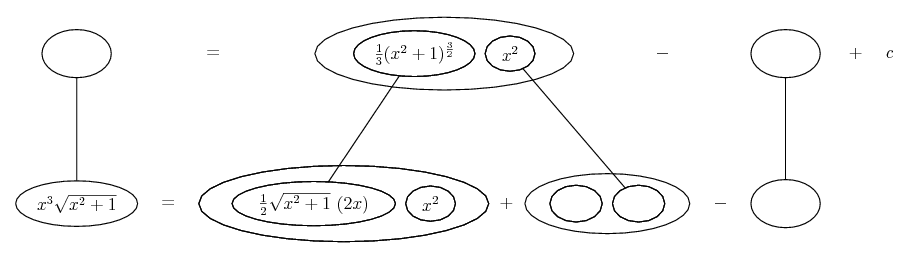But let's zoom in on the anti-differentiation...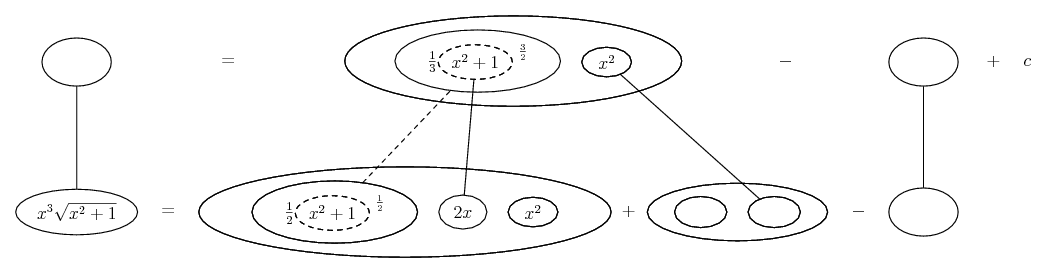...where... is the chain rule. Straight continuous lines still differentiate downwards (anti-diff up) with respect to x, and the straight dashed line similarly but with respect to the dashed balloon expression (the inner function of the composite which is subject to the chain rule).

Spoiler: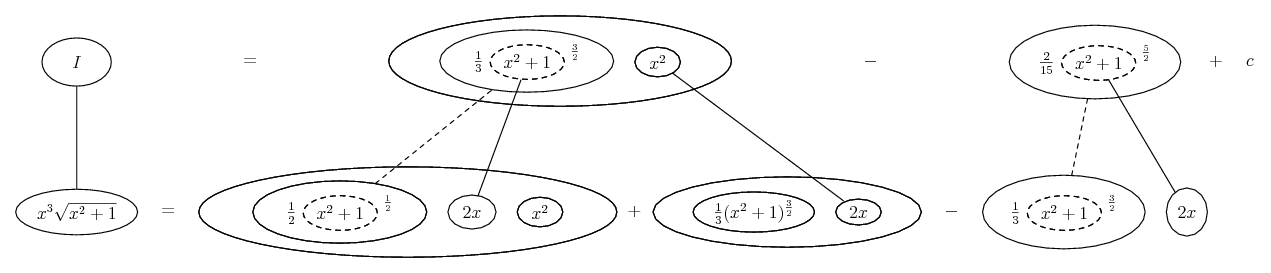Which is also, though less obviously, equivalent to the previous answers given.

_________________________________________
Don't integrate - balloontegrate!

Balloon Calculus; standard integrals, derivatives and methods

Balloon Calculus Drawing with LaTeX and Asymptote!

#### Search Tags

integration, substitution